login  home  contents  what's new  discussion  bug reports help  links  subscribe  changes  refresh  edit

Quaternion Algebra Is Frobenius In Many Ways

Linear operators over a 4-dimensional vector space representing quaternion algebra

Ref:

We need the Axiom LinearOperator library.

fricas
)library CARTEN ARITY CMONAL CPROP CLOP CALEY
CartesianTensor is now explicitly exposed in frame initial
CartesianTensor will be automatically loaded when needed from
/var/aw/var/LatexWiki/CARTEN.NRLIB/CARTEN
Arity is now explicitly exposed in frame initial
Arity will be automatically loaded when needed from
/var/aw/var/LatexWiki/ARITY.NRLIB/ARITY
ClosedMonoidal is now explicitly exposed in frame initial
ClosedMonoidal will be automatically loaded when needed from
/var/aw/var/LatexWiki/CMONAL.NRLIB/CMONAL
ClosedProp is now explicitly exposed in frame initial
ClosedProp will be automatically loaded when needed from
/var/aw/var/LatexWiki/CPROP.NRLIB/CPROP
ClosedLinearOperator is now explicitly exposed in frame initial
ClosedLinearOperator will be automatically loaded when needed from
/var/aw/var/LatexWiki/CLOP.NRLIB/CLOP
CaleyDickson is now explicitly exposed in frame initial
CaleyDickson will be automatically loaded when needed from
/var/aw/var/LatexWiki/CALEY.NRLIB/CALEY

Use the following macros for convenient notation

fricas
-- summation
macro Σ(x,i,n)==reduce(+,[x for i in n])
Type: Void
fricas
-- subscript and superscripts
macro sb == subscript
Type: Void
fricas
macro sp == superscript
Type: Void

ℒ is the domain of 4-dimensional linear operators over the rational functions ℚ (Expression Integer), i.e. ratio of polynomials with integer coefficients.

fricas
dim:=4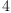(1)
Type: PositiveInteger?
fricas
macro ℂ == CaleyDickson
Type: Void
fricas
macro ℚ == Expression Integer
Type: Void
fricas
ℒ := ClosedLinearOperator(OVAR ['1,'i,'j,'k], ℚ)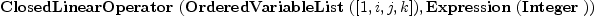(2)
Type: Type
fricas
ⅇ:List ℒ      := basisOut()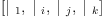(3)
Type: List(ClosedLinearOperator(OrderedVariableList([1,i,j,k]),Expression(Integer)))
fricas
ⅆ:List ℒ      := basisIn()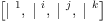(4)
Type: List(ClosedLinearOperator(OrderedVariableList([1,i,j,k]),Expression(Integer)))
fricas
I:ℒ:=   -- identity for composition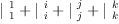(5)
Type: ClosedLinearOperator(OrderedVariableList([1,i,j,k]),Expression(Integer))
fricas
X:ℒ:=[2,1] -- twist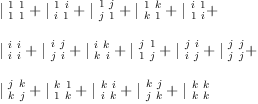(6)
Type: ClosedLinearOperator(OrderedVariableList([1,i,j,k]),Expression(Integer))
fricas
V:ℒ:=ev(1) -- evaluation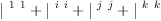(7)
Type: ClosedLinearOperator(OrderedVariableList([1,i,j,k]),Expression(Integer))
fricas
Λ:ℒ:=co(1) -- co-evaluation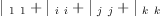(8)
Type: ClosedLinearOperator(OrderedVariableList([1,i,j,k]),Expression(Integer))
fricas
equate(eq)==map((x,y)+->(x=y),ravel lhs eq, ravel rhs eq);
Type: Void

Now generate structure constants for Quaternion Algebra

The basis consists of the real and imaginary units. We use quaternion multiplication to form the "multiplication table" as a matrix. Then the structure constants can be obtained by dividing each matrix entry by the list of basis vectors.

Split-complex and co-quaternions can be specified by Caley-Dickson parameters (i2, j2)

fricas
i2:=sp('i,)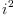(9)
Type: Symbol
fricas
--i2:= -1  -- complex
j2:=sp('j,)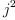(10)
Type: Symbol
fricas
--j2:= -1  -- quaternion
k2:=-i2*j2;
Type: Polynomial(Integer)
fricas
QQ := ℂ(ℂ(ℚ,'i,-i2),'j,-j2);
Type: Type

Basis: Each B.i is a quaternion number

fricas
B:List QQ := map(x +-> hyper x,1$SQMATRIX(dim,ℚ)::List List ℚ)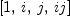(11) Type: List(CaleyDickson(CaleyDickson(Expression(Integer),i,-(i[;2])),j,-(j[;2]))) fricas -- Multiplication table: M:Matrix QQ := matrix [[B.i*B.j for i in 1..dim] for j in 1..dim]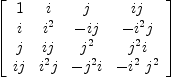(12) Type: Matrix(CaleyDickson(CaleyDickson(Expression(Integer),i,-(i[;2])),j,-(j[;2]))) fricas -- Function to divide the matrix entries by a basis element S(y) == map(x +-> real real(x/y),M) Type: Void fricas -- The result is a nested list ѕ :=map(S,B)::List List List ℚ fricas Compiling function S with type CaleyDickson(CaleyDickson(Expression( Integer),i,-(i[;2])),j,-(j[;2])) -> Matrix(Expression(Integer))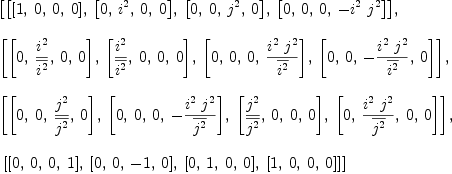(13) Type: List(List(List(Expression(Integer)))) fricas -- structure constants form a tensor operator Y := Σ(Σ(Σ(ѕ(i)(k)(j)*ⅇ.i*ⅆ.j*ⅆ.k, i,1..dim), j,1..dim), k,1..dim)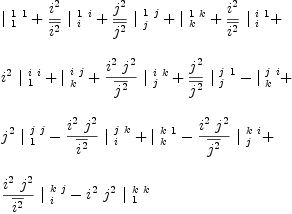(14) Type: ClosedLinearOperator(OrderedVariableList([1,i,j,k]),Expression(Integer)) fricas arity Y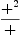(15) Type: ClosedProp?(ClosedLinearOperator(OrderedVariableList([1,i,j,k]),Expression(Integer))) fricas matrix [[(ⅇ.i*ⅇ.j)/Y for i in 1..dim] for j in 1..dim]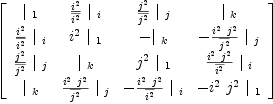(16) Type: Matrix(ClosedLinearOperator(OrderedVariableList([1,i,j,k]),Expression(Integer))) Units fricas q:=ⅇ.1; i:=ⅇ.2; j:=ⅇ.3; k:=ⅇ.4; Type: ClosedLinearOperator(OrderedVariableList([1,i,j,k]),Expression(Integer)) Multiplication of arbitrary quaternions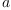and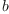fricas a:=Σ(sb('a,[i])*ⅇ.i, i,1..dim)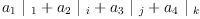(17) Type: ClosedLinearOperator(OrderedVariableList([1,i,j,k]),Expression(Integer)) fricas b:=Σ(sb('b,[i])*ⅇ.i, i,1..dim)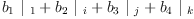(18) Type: ClosedLinearOperator(OrderedVariableList([1,i,j,k]),Expression(Integer)) fricas (a*b)/Y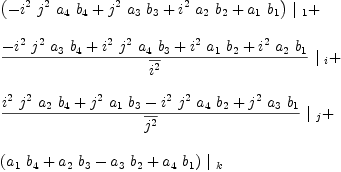(19) Type: ClosedLinearOperator(OrderedVariableList([1,i,j,k]),Expression(Integer)) Multiplication is Associative fricas test( ( I Y ) / _ ( Y ) = _ ( Y I ) / _ ( Y ) )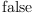(20) Type: Boolean A scalar product is denoted by the (2,0)-tensor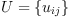fricas U:=Σ(Σ(script('u,[[],[i,j]])*ⅆ.i*ⅆ.j, i,1..dim), j,1..dim)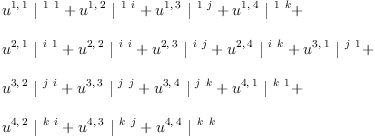(21) Type: ClosedLinearOperator(OrderedVariableList([1,i,j,k]),Expression(Integer)) ## Definition 1 We say that the scalar product is associative if the tensor equation holds:  Y = Y U U  In other words, if the (3,0)-tensor: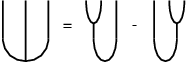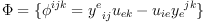(22) (three-point function) is zero. Using the LinearOperator domain in Axiom and some carefully chosen symbols we can easily enter expressions that are both readable and interpreted by Axiom as "graphical calculus" diagrams describing complex products and compositions of linear operators. fricas ω:ℒ := ( Y I ) / U - ( I Y ) / U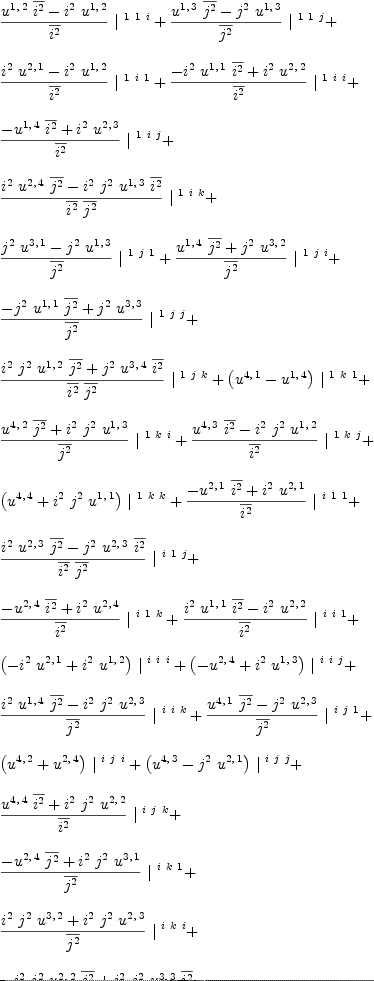(23) Type: ClosedLinearOperator(OrderedVariableList([1,i,j,k]),Expression(Integer)) ## Definition 2 An algebra with a non-degenerate associative scalar product is called a [Frobenius Algebra]?. The Cartan-Killing Trace fricas Ú:= ( Y Λ ) / _ ( Y I ) / _ V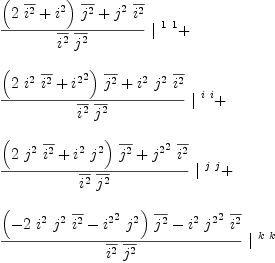(24) Type: ClosedLinearOperator(OrderedVariableList([1,i,j,k]),Expression(Integer)) fricas Ù:= ( Λ Y ) / _ ( I Y ) / _ V(25) Type: ClosedLinearOperator(OrderedVariableList([1,i,j,k]),Expression(Integer)) fricas test(Ù=Ú)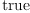(26) Type: Boolean forms a non-degenerate associative scalar product for Y fricas Ũ := Ù(27) Type: ClosedLinearOperator(OrderedVariableList([1,i,j,k]),Expression(Integer)) fricas test ( Y I ) / Ũ = ( I Y ) / Ũ(28) Type: Boolean fricas determinant [[retract((ⅇ.i * ⅇ.j)/Ũ) for j in 1..dim] for i in 1..dim]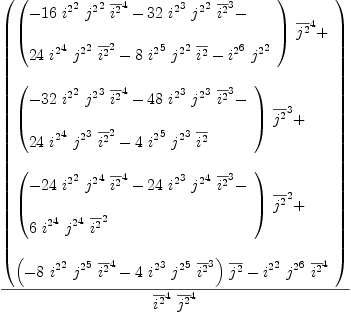(29) Type: Expression(Integer) General Solution We may consider the problem where multiplication Y is given, and look for all associative scalar products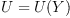This problem can be solved using linear algebra. fricas )expose MCALCFN MultiVariableCalculusFunctions is now explicitly exposed in frame initial J := jacobian(ravel ω,concat map(variables,ravel U)::List Symbol); Type: Matrix(Expression(Integer)) fricas nrows(J),ncols(J)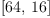(30) Type: Tuple(PositiveInteger?) The matrix J transforms the coefficients of the tensor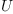into coefficients of the tensor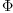. We are looking for the general linear family of tensors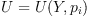such that J transformsinto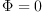for any such. If the null space of the J matrix is not empty we can use the basis to find all non-trivial solutions for U: fricas Ñ:=nullSpace(J)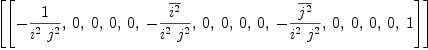(31) Type: List(Vector(Expression(Integer))) fricas ℰ:=map((x,y)+->x=y, concat map(variables,ravel U), entries Σ(sb('p,[i])*Ñ.i, i,1..#Ñ) )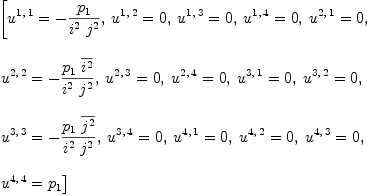(32) Type: List(Equation(Expression(Integer))) This defines a family of pre-Frobenius algebras: fricas zero? eval(ω,ℰ)(33) Type: Boolean fricas Ų:ℒ := eval(U,ℰ)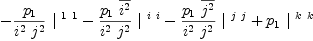(34) Type: ClosedLinearOperator(OrderedVariableList([1,i,j,k]),Expression(Integer)) Frobenius Form (co-unit) fricas d:=ε1*ⅆ.1+εi*ⅆ.2+εj*ⅆ.3+εk*ⅆ.4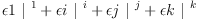(35) Type: ClosedLinearOperator(OrderedVariableList([1,i,j,k]),Expression(Integer)) fricas equate(d= ( q I ) / _ Ų ) fricas Compiling function equate with type Equation(ClosedLinearOperator( OrderedVariableList([1,i,j,k]),Expression(Integer))) -> List( Equation(Expression(Integer)))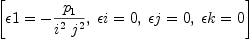(36) Type: List(Equation(Expression(Integer))) Express scalar product in terms of Frobenius form fricas Ξ:=solve(%,[sb('p,[i]) for i in 1..#Ñ]).1 >> System error: Cannot take first of an empty list In general the pairing is not symmetric! fricas u1:=matrix [[retract((ⅇ.i ⅇ.j)/Ų) for i in 1..dim] for j in 1..dim]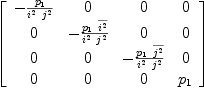(37) Type: Matrix(Expression(Integer)) The scalar product must be non-degenerate: fricas Ů:=determinant u1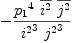(38) Type: Expression(Integer) fricas factor(numer Ů)/factor(denom Ů)(39) Type: Fraction(Factored(SparseMultivariatePolynomial?(Integer,Kernel(Expression(Integer))))) Cartan-Killing is a special case fricas ck:=solve(equate(eval(Ũ,Ξ)=Ų),[ε1,εi,εj,εk]).1 There are 12 exposed and 6 unexposed library operations named eval having 2 argument(s) but none was determined to be applicable. Use HyperDoc Browse, or issue )display op eval to learn more about the available operations. Perhaps package-calling the operation or using coercions on the arguments will allow you to apply the operation. Cannot find a definition or applicable library operation named eval with argument type(s) ClosedLinearOperator(OrderedVariableList([1,i,j,k]),Expression(Integer)) Variable(Ξ) Perhaps you should use "@" to indicate the required return type, or "$" to specify which version of the function you need.

Frobenius scalar product of "vector" quaternionsandfricas
a:=sb('a,)*i+sb('a,)*j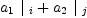(40)
Type: ClosedLinearOperator(OrderedVariableList([1,i,j,k]),Expression(Integer))
fricas
b:=sb('b,)*i+sb('b,)*j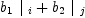(41)
Type: ClosedLinearOperator(OrderedVariableList([1,i,j,k]),Expression(Integer))
fricas
(a,a)/Ų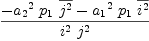(42)
Type: ClosedLinearOperator(OrderedVariableList([1,i,j,k]),Expression(Integer))
fricas
(b,b)/Ų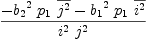(43)
Type: ClosedLinearOperator(OrderedVariableList([1,i,j,k]),Expression(Integer))
fricas
(a,b)/Ų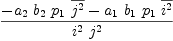(44)
Type: ClosedLinearOperator(OrderedVariableList([1,i,j,k]),Expression(Integer))

## Definition 3

Co-scalar product

Solve the Snake Relation as a system of linear equations.

fricas
Ω:ℒ:=Σ(Σ(script('u,[[i,j]])*ⅇ.i*ⅇ.j, i,1..dim), j,1..dim)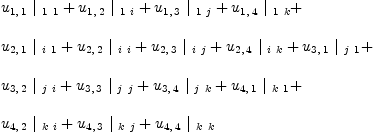(45)
Type: ClosedLinearOperator(OrderedVariableList([1,i,j,k]),Expression(Integer))
fricas
ΩX:=Ω/X;
Type: ClosedLinearOperator(OrderedVariableList([1,i,j,k]),Expression(Integer))
fricas
UXΩ:=(I*ΩX)/(Ų*I);
Type: ClosedLinearOperator(OrderedVariableList([1,i,j,k]),Expression(Integer))
fricas
ΩXU:=(ΩX*I)/(I*Ų);
Type: ClosedLinearOperator(OrderedVariableList([1,i,j,k]),Expression(Integer))
fricas
eq1:=equate(UXΩ=I);
Type: List(Equation(Expression(Integer)))
fricas
eq2:=equate(ΩXU=I);
Type: List(Equation(Expression(Integer)))
fricas
snake:=solve(concat(eq1,eq2),concat [[script('u,[[i,j]]) for i in 1..dim] for j in 1..dim]);
Type: List(List(Equation(Expression(Integer))))
fricas
if #snake ~= 1 then error "no solution"
Type: Void
fricas
Ω:=eval(Ω,snake(1))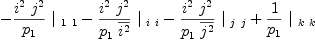(46)
Type: ClosedLinearOperator(OrderedVariableList([1,i,j,k]),Expression(Integer))
fricas
ΩX:=Ω/X;
Type: ClosedLinearOperator(OrderedVariableList([1,i,j,k]),Expression(Integer))

The common demoninator is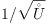fricas
squareFreePart factor denom Ů / squareFreePart factor numer Ů
Function:  squareFree : % -> Factored(%) is missing from domain: Factored(SparseMultivariatePolynomial(Integer,Kernel(Expression(Integer))))
Internal Error
The function squareFree with signature (Factored $)$ is missing from
domain Factored
(SparseMultivariatePolynomial (Integer) (Kernel (Expression (Integer))))

Check "dimension" and the snake relations.

fricas
O:ℒ:=
Ω    /
Ų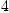(47)
Type: ClosedLinearOperator(OrderedVariableList([1,i,j,k]),Expression(Integer))
fricas
test
(    I ΩX     )  /
(     Ų I     )  =  I(48)
Type: Boolean
fricas
test
(     ΩX I    )  /
(    I Ų      )  =  I(49)
Type: Boolean

Cartan-Killing co-scalar

fricas
eval(Ω,ck)
There are 12 exposed and 6 unexposed library operations named eval
having 2 argument(s) but none was determined to be applicable.
Use HyperDoc Browse, or issue
)display op eval
package-calling the operation or using coercions on the arguments
will allow you to apply the operation.
Cannot find a definition or applicable library operation named eval
with argument type(s)
ClosedLinearOperator(OrderedVariableList([1,i,j,k]),Expression(Integer))
Variable(ck)
Perhaps you should use "@" to indicate the required return type,
or "$" to specify which version of the function you need. ## Definition 4 Co-algebra Compute the "three-point" function and use it to define co-multiplication. fricas W:= (Y I) / Ų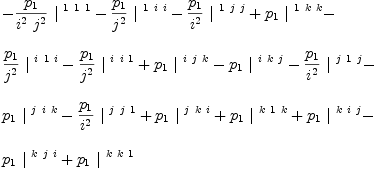(50) Type: ClosedLinearOperator(OrderedVariableList([1,i,j,k]),Expression(Integer)) fricas λ:= ( ΩX I ΩX ) / ( I W I )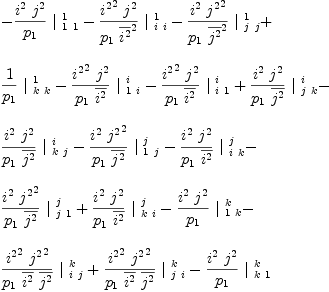(51) Type: ClosedLinearOperator(OrderedVariableList([1,i,j,k]),Expression(Integer)) Cartan-Killing co-multiplication fricas eval(λ,ck) There are 12 exposed and 6 unexposed library operations named eval having 2 argument(s) but none was determined to be applicable. Use HyperDoc Browse, or issue )display op eval to learn more about the available operations. Perhaps package-calling the operation or using coercions on the arguments will allow you to apply the operation. Cannot find a definition or applicable library operation named eval with argument type(s) ClosedLinearOperator(OrderedVariableList([1,i,j,k]),Expression(Integer)) Variable(ck) Perhaps you should use "@" to indicate the required return type, or "$" to specify which version of the function you need.

fricas
test
(    I ΩX     )  /
(     Y I     )  =  λ(52)
Type: Boolean
fricas
test
(     ΩX I    )  /
(    I  Y     )  =  λ(53)
Type: Boolean

Co-associativity

fricas
test(
(  λ  ) / _
( I λ ) = _
(  λ  ) / _
( λ I ) )(54)
Type: Boolean

fricas
test
q     /
λ     =    ΩX(55)
Type: Boolean

Frobenius Condition (fork)

fricas
H :=
Y    /
λ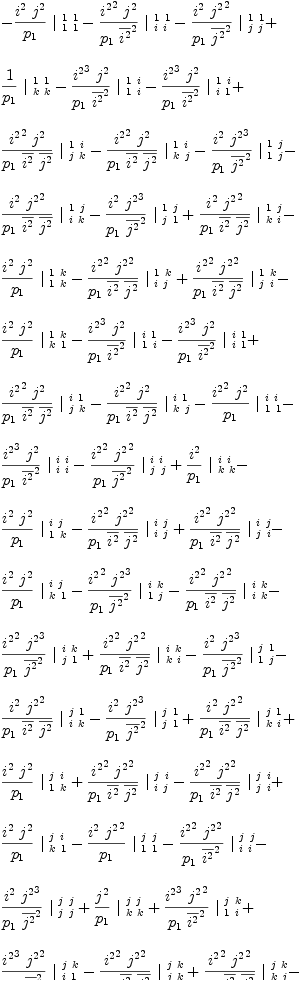(56)
Type: ClosedLinearOperator(OrderedVariableList([1,i,j,k]),Expression(Integer))
fricas
test
(   λ I   )  /
(  I Y    )  =  H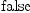(57)
Type: Boolean
fricas
test
(   I λ   )  /
(    Y I  )  =  H(58)
Type: Boolean

The Cartan-Killing form makes H of the Frobenius condition idempotent

fricas
test( eval(H,ck)=eval(H/H,ck) )
There are 12 exposed and 6 unexposed library operations named eval
having 2 argument(s) but none was determined to be applicable.
Use HyperDoc Browse, or issue
)display op eval
package-calling the operation or using coercions on the arguments
will allow you to apply the operation.
Cannot find a definition or applicable library operation named eval
with argument type(s)
ClosedLinearOperator(OrderedVariableList([1,i,j,k]),Expression(Integer))
Variable(ck)
Perhaps you should use "@" to indicate the required return type,
or "$" to specify which version of the function you need. But it is not unique. E.g. other idempots fricas h1:=map(numer,ravel(H-H/H)::List FRAC POLY INT); Cannot convert the value from type List(Expression(Integer)) to List (Fraction(Polynomial(Integer))) . Handle and handle element fricas Φ := λ / Y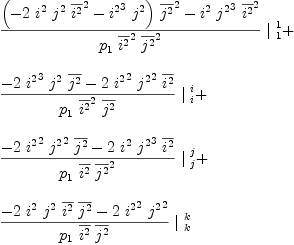(59) Type: ClosedLinearOperator(OrderedVariableList([1,i,j,k]),Expression(Integer)) fricas Φ1 := q / Φ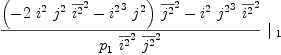(60) Type: ClosedLinearOperator(OrderedVariableList([1,i,j,k]),Expression(Integer)) The Cartan-Killing form makes Φ into the identity fricas test( eval(Φ,ck)=I ) There are 12 exposed and 6 unexposed library operations named eval having 2 argument(s) but none was determined to be applicable. Use HyperDoc Browse, or issue )display op eval to learn more about the available operations. Perhaps package-calling the operation or using coercions on the arguments will allow you to apply the operation. Cannot find a definition or applicable library operation named eval with argument type(s) ClosedLinearOperator(OrderedVariableList([1,i,j,k]),Expression(Integer)) Variable(ck) Perhaps you should use "@" to indicate the required return type, or "$" to specify which version of the function you need.

but it can be the identity in many ways. For example,

fricas
solve(equate(eval(Φ,[ε1=1,εi=1,εj=1,j2=-1])=I),[εk])(61)
Type: List(List(Equation(Expression(Integer))))

If handle is identity then fork is idempotent but the converse is not true

fricas
Φ1:=map(numer,ravel(Φ-I)::List FRAC POLY INT);
Cannot convert the value from type List(Expression(Integer)) to List
(Fraction(Polynomial(Integer))) .

Figure 12

fricas
φφ:=          _
( Ω  Ω  ) / _
( X I I ) / _
( I X I ) / _
( I I X ) / _
(  Y  Y );
Type: ClosedLinearOperator(OrderedVariableList([1,i,j,k]),Expression(Integer))
fricas
φφ1:=map((x:ℚ):ℚ+->numer x,φφ)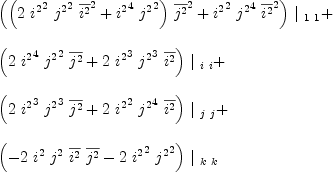(62)
Type: ClosedLinearOperator(OrderedVariableList([1,i,j,k]),Expression(Integer))
fricas
φφ2:=denom(ravel(φφ).1)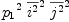(63)
Type: SparseMultivariatePolynomial?(Integer,Kernel(Expression(Integer)))
fricas
test(φφ=(1/φφ2)*φφ1)(64)
Type: Boolean

For Cartan-Killing this is just the co-scalar

fricas
test(eval(φφ,ck)=eval(Ω,ck))
There are 12 exposed and 6 unexposed library operations named eval
having 2 argument(s) but none was determined to be applicable.
Use HyperDoc Browse, or issue
)display op eval
package-calling the operation or using coercions on the arguments
will allow you to apply the operation.
Cannot find a definition or applicable library operation named eval
with argument type(s)
ClosedLinearOperator(OrderedVariableList([1,i,j,k]),Expression(Integer))
Variable(ck)
Perhaps you should use "@" to indicate the required return type,
or "$" to specify which version of the function you need. Bi-algebra conditions fricas ΦΦ:= _ ( λ λ ) / _ ( I I X ) / _ ( I X I ) / _ ( I I X ) / _ ( Y Y ) ; Type: ClosedLinearOperator(OrderedVariableList([1,i,j,k]),Expression(Integer)) fricas test((q,q)/ΦΦ=φφ)(65) Type: Boolean fricas test(eval(ΦΦ,ck)=eval(H,ck)) There are 12 exposed and 6 unexposed library operations named eval having 2 argument(s) but none was determined to be applicable. Use HyperDoc Browse, or issue )display op eval to learn more about the available operations. Perhaps package-calling the operation or using coercions on the arguments will allow you to apply the operation. Cannot find a definition or applicable library operation named eval with argument type(s) ClosedLinearOperator(OrderedVariableList([1,i,j,k]),Expression(Integer)) Variable(ck) Perhaps you should use "@" to indicate the required return type, or "$" to specify which version of the function you need.

Theorem 8.3

fricas
u2:=map(retract,matrix [ _
[q/d,     i/d,    j/d,     k/d], _
[i/d,  i2*q/d,    k/d,  i2*j/d], _
[j/d,    -k/d, j2*q/d, -j2*i/d], _
[k/d, -i2*j/d, j2*i/d, k2*q/d]])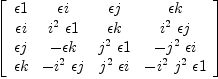(66)
Type: Matrix(Expression(Integer))
fricas
test(u2=transpose(u1))(67)
Type: Boolean
fricas
Ů2 := -retract( k2*(q/d)^2 + j2*(i/d)^2 + i2*(j/d)^2 - (k/d)^2 )^2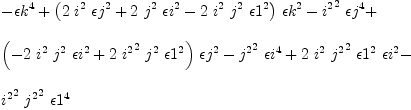(68)
Type: Expression(Integer)
fricas
factor(numer Ů2)/factor(denom Ů2)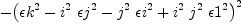(69)
Type: Fraction(Factored(SparseMultivariatePolynomial?(Integer,Kernel(Expression(Integer)))))
fricas
test(Ů=Ů2)(70)
Type: Boolean

 Subject:   Be Bold !! ( 14 subscribers )